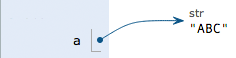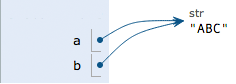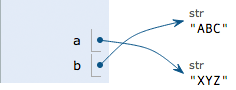> Python3中文手册 > 数据类型和变量

# 数据类型和变量

## 数据类型

### 整数

Python可以处理任意大小的整数，当然包括负整数，在程序中的表示方法和数学上的写法一模一样，例如：`1``100``-8080``0`，等等。

### 字符串

``'I\'m \"OK\"!'``

``I'm "OK"!``

``````>>> print('I\'m ok.')
I'm ok.
>>> print('I\'m learning\nPython.')
I'm learning
Python.
>>> print('\\\n\\')
\
\``````

``````>>> print('\\\t\\')
\       \
>>> print(r'\\\t\\')
\\\t\\``````

``````>>> print('''line1
... line2
... line3''')
line1
line2
line3``````

``````print('''line1
line2
line3''')``````

### 布尔值

``````>>> True
True
>>> False
False
>>> 3 > 2
True
>>> 3 > 5
False``````

`and`运算是与运算，只有所有都为`True``and`运算结果才是`True`

``````>>> True and True
True
>>> True and False
False
>>> False and False
False
>>> 5 > 3 and 3 > 1
True``````

`or`运算是或运算，只要其中有一个为`True``or`运算结果就是`True`

``````>>> True or True
True
>>> True or False
True
>>> False or False
False
>>> 5 > 3 or 1 > 3
True``````

`not`运算是非运算，它是一个单目运算符，把`True`变成`False``False`变成`True`

``````>>> not True
False
>>> not False
True
>>> not 1 > 2
True``````

``````if age >= 18:
else:
print('teenager')``````

## 变量

``a = 1``

``t_007 = 'T007'``

``Answer = True``

``````a = 123 # a是整数
print(a)
a = 'ABC' # a变为字符串
print(a)``````

``````int a = 123; // a是整数类型变量
a = "ABC"; // 错误：不能把字符串赋给整型变量``````

``````x = 10
x = x + 2``````

``a = 'ABC'``

1. 在内存中创建了一个`'ABC'`的字符串；

2. 在内存中创建了一个名为`a`的变量，并把它指向`'ABC'`

``````a = 'ABC'
b = a
a = 'XYZ'
print(b)``````## 常量

``PI = 3.14159265359``

``````>>> 10 / 3
3.3333333333333335``````

`/`除法计算结果是浮点数，即使是两个整数恰好整除，结果也是浮点数：

``````>>> 9 / 3
3.0``````

``````>>> 10 // 3
3``````

``````>>> 10 % 3
1``````

## 练习

``````n = 123
f = 456.789
s1 = 'Hello, world'
s3 = r'Hello, "Bart"'
s4 = r'''Hello,
Lisa!'''``````

## 小结

Python支持多种数据类型，在计算机内部，可以把任何数据都看成一个“对象”，而变量就是在程序中用来指向这些数据对象的，对变量赋值就是把数据和变量给关联起来。

Python的浮点数也没有大小限制，但是超出一定范围就直接表示为`inf`（无限大）。